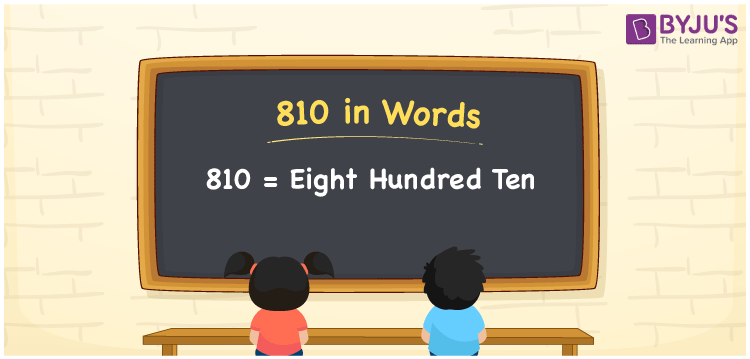# 810 in Words

The number 810 in words is Eight Hundred Ten. The number 810 has three digits and the number name of 810 can be written using the place value system. In mathematics, 810 is a natural number and it is also considered to be a cardinal number. For example, the cost of 5 kg apples is Rs. 810. Here, we have included the place value table of 810 and its procedure in detail.

 810 in Words: Eight Hundred Ten. Eight Hundred Ten in Numerical Form: 810.

## 810 in English Words## How to Write 810 in Words?

The place value table for the number 810 is presented below:

 Hundreds Tens Ones 8 1 0

The expanded form of 810 is as follows:

= 8 × Hundred + 1 × Ten + 0 × One

= 8 × 100 + 1 × 10 + 0 × 1

= 800 + 10

= 810

= eight hundred ten

Hence, 810 in words is eight hundred ten.

The number 810 appears between 809 and 811.

810 in words – Eight hundred ten

Is 810 an odd number? – No

Is 810 an even number? – Yes

Is 810 a perfect square number? – No

Is 810 a perfect cube number? – No

Is 810 a prime number? – No

Is 810 a composite number? – Yes

## Frequently Asked Questions on 810 in Words

Q1

### How to write 810 in words?

810 in words is eight hundred ten.

Q2

### Simplify 800 + 10, and express it in words.

Simplifying 800 + 10, we get 810. Hence, 810 in words is eight hundred ten.

Q3

### Is 810 an odd number?

No, 810 is not an odd number.